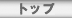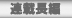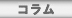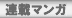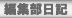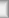# \$B4Q;!\$5\$l\$k\$b\$N\$H5-O?\$5\$l\$J\$\$\$b\$N(J

The person who is not recorded with an observed person

## \$B\$?\$J\$+\$J\$D\$_(J

\$B!!%"%K%^%=%i%j%9#7<~G/5-G09f\$K!"#1G/\$V\$j\$K!V\$?\$J\$+\$J\$D\$_!W@h@8\$NEP>l\$G\$9!#(J
\$B!!eLL)\$JI=8=\$H?4\$K9o\$`\$h\$&\$J8@MU\$K:L\$i\$l\$?G;L)\$J@\$3&\$r\$*3Z\$7\$_\$/\$@\$5\$\$!#(J

\$B7G:\9f(J \$B%?%\$%H%k(J HTML
\$BBh#8#49f(J \$B4Q;!\$5\$l\$k\$b\$N\$H5-O?\$5\$l\$J\$\$\$b\$N(J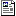17KB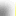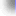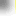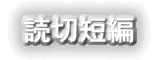\$B%H%C%W(J\$B!?(J\$BFI@ZC;JT(J\$B!?(J\$BO":\D9JT(J\$B!?(J\$B%3%i%`(J
\$B%V%C%/%l%S%e!<(J\$B!?(J\$BCx\$B!?(J\$BO":\%^%s%,(J\$B!?(JBBS\$B!?(J\$BJT=8ItF|5-(J
\$BCx:n8"(J\$B!?(J\$B%W%i%\$%P%7!<%]%j%7!<(J\$B!?(J\$B%5%\$%H%^%C%W(J